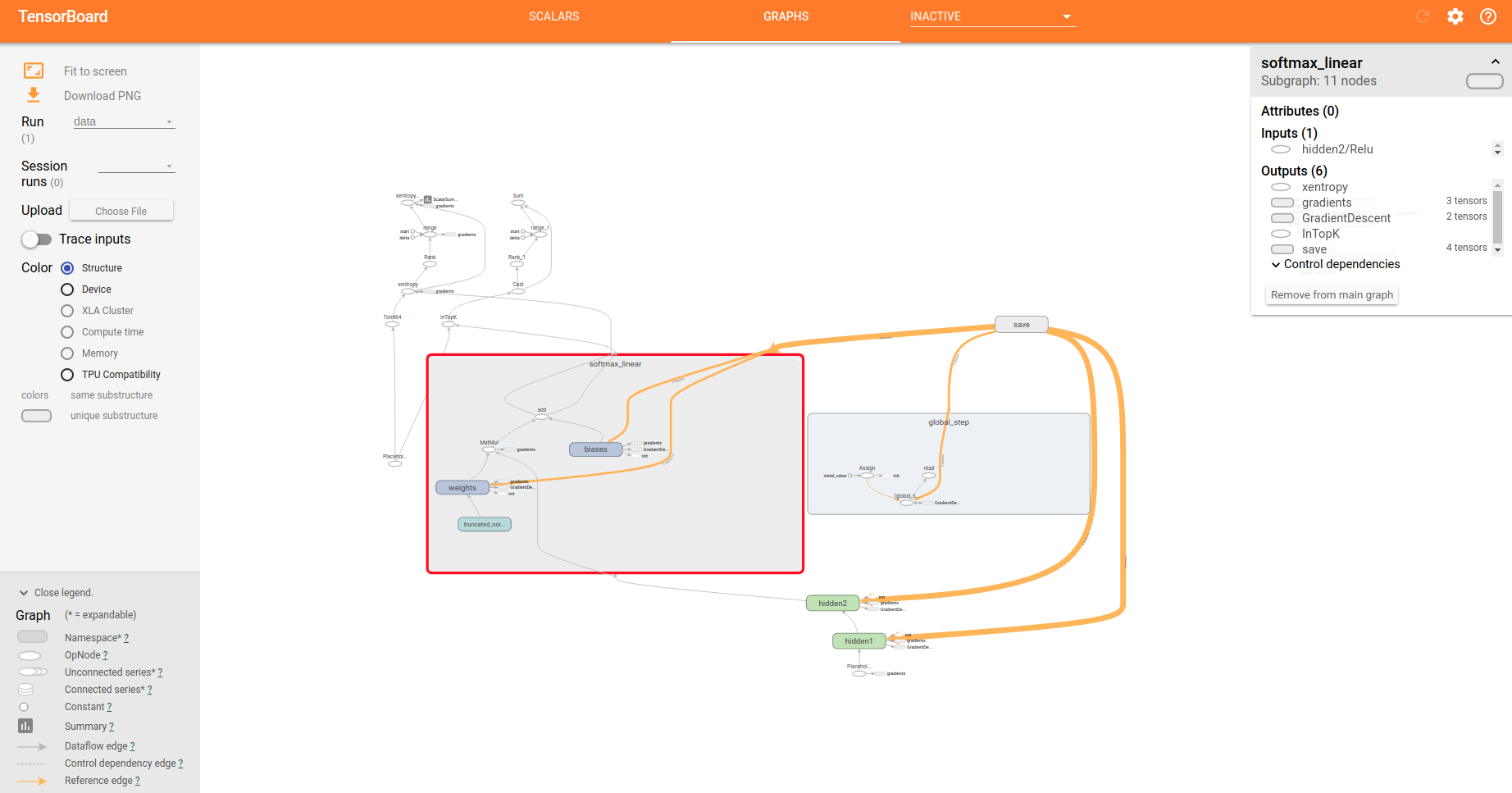﻿

# 文章结构

1. TensorFlow相关介绍
2. TensorFlow的安装使用(入门级)

# 1.关于TensorFlow

## 1.1什么是数据流图（Data Flow Graph）?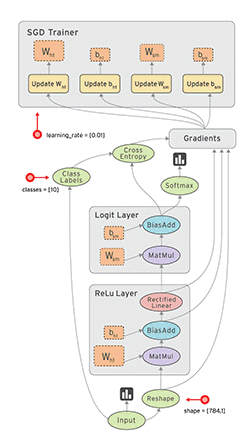## 1.2TensorFLow 六大特性

1.高度的灵活性—-提供很多的工具让你来构建图,也可以在tf基础上写上层库,对tf操作进行组合,还可以动手丰富底层操作,自己添加tf内容
2.真正的可移植性—-tf在CPU和GPU上运行，可以运行在台式机、服务器、手机移动设备,Android,ios都可以,平台之间转移可以不用改
3.将科研和产品联系在一起—-tf可以免去很大的代码重写工作,帮助科研工作者提高科研产出率
4.自动求微分—-用户只需要定义预测模型的结构，将这个结构和目标函数结合在一起，并添加数据,tf将自动为你计算相关的微分导数
5.多语言支持—-官方文档中写明,目前有python/c++使用界面,还鼓励开发者开发其他语言
6.性能最优化—-给予了线程、队列、异步操作等以最佳支持,tf让你可以将你手边硬件的计算潜能全部发挥出来。你可以自由地将tf图中的计算元素分配到不同设备上,tf可以帮你管理好这些不同副本

## 1.3TensorFLow三个有趣的应用案例

1.皮肤癌图像分类2.利用AI预测糖尿病，预防失明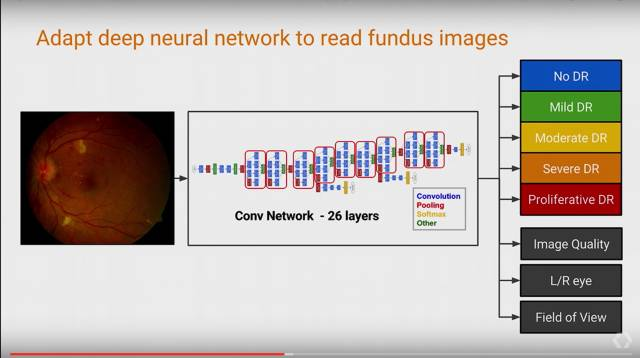3.自动控制数据中心冷却设备# 2.TensroFlow的安装使用

## 2.1TensorFlow的安装

### 2.1.1相关依赖包安装

TensorFlow的python API依赖python2.7版本,所以我们要先安装python以及pip.

``````#python安装
sudo apt-get install python2.7 python2.7-dev
#pip安装
sudo apt-get install python-pip python-dev build-essential
``````

### 2.1.2TensorFlow安装

TensorFlow有cpu版本跟gpu版本的安装,但是我们的电脑没有独显,所以为了让我们可以继续学习如何使用,我们安装cpu版本的.

``````sudo pip install ./tensorflow-0.5.0-cp27-none-linux_x86_64.whl
``````

### 2.1.3测试安装是否成功``````>>> import tensorflow as tf #tensorflow是API,需要导入包
>>> hello = tf.constant('hello,TF') #创建一个hello常量
>>> sess = tf.Sesssion()
>>> print sess.run(hello)#要使用seesion才可以真正实例化对象
>>> #后面的代码就不多解释了
``````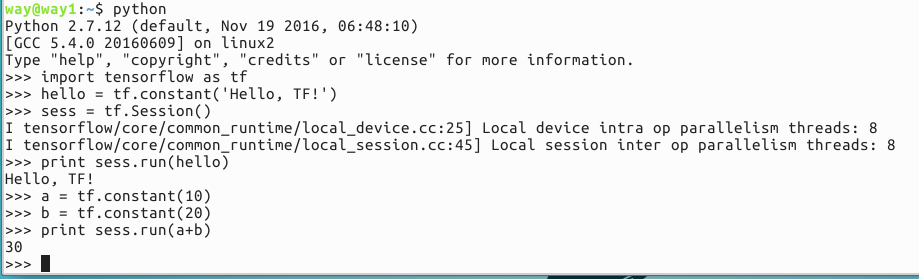## 2.2TensorFlow的使用—MNIST初级入门

MNIST是一个入门级的计算机视觉数据集，它包含如下各种手写数字图片:### 2.2.1下载数据集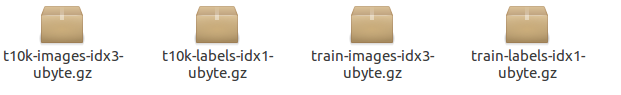2.下载好的文件本身并没有使用标准的图片格式储存，并且需要使用input_data.py文件中extract_images() 和extract_labels()函数来手动解压.图片数据将被解压成2维的tensor：[image index, pixel index], “image index”代表数据集中图片的编号, 从0到数据集的上限值。”pixel index”代表该图片中像素点得个数, 从0到图片的像素上限值。以train-*开头的文件中包括60000个样本，其中分割出55000个样本作为训练集，其余的5000个样本作为验证集。因为所有数据集中28×28像素的灰度图片的尺寸为784，所以训练集输出的tensor格式为[55000, 784]。

data_sets.train 55000 组 图片和标签, 用于训练。
data_sets.validation 5000 组 图片和标签, 用于迭代验证训练的准确性。
data_sets.test 10000 组 图片和标签, 用于最终测试训练的准确性。

images_feed, labels_feed = data_set.next_batch(FLAGS.batch_size)

### input_data.py的使用方法

``````import input_data
``````

### 2.2.2数据集介绍## 2.3softmax模型介绍

softmax回归是要对一个未知对象进行预测的概率分配模型.也就是说,输入一个图片x,softmax会得到x分别是0,1,2..的概率.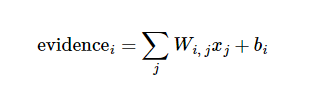softmax可以把我们定义的线性函数的输出转换成我们想要的格式，也就是关于10个数字类的概率分布。因此，给定一张图片，它对于每一个数字的吻合度可以被softmax函数转换成为一个概率值。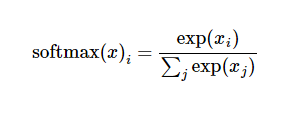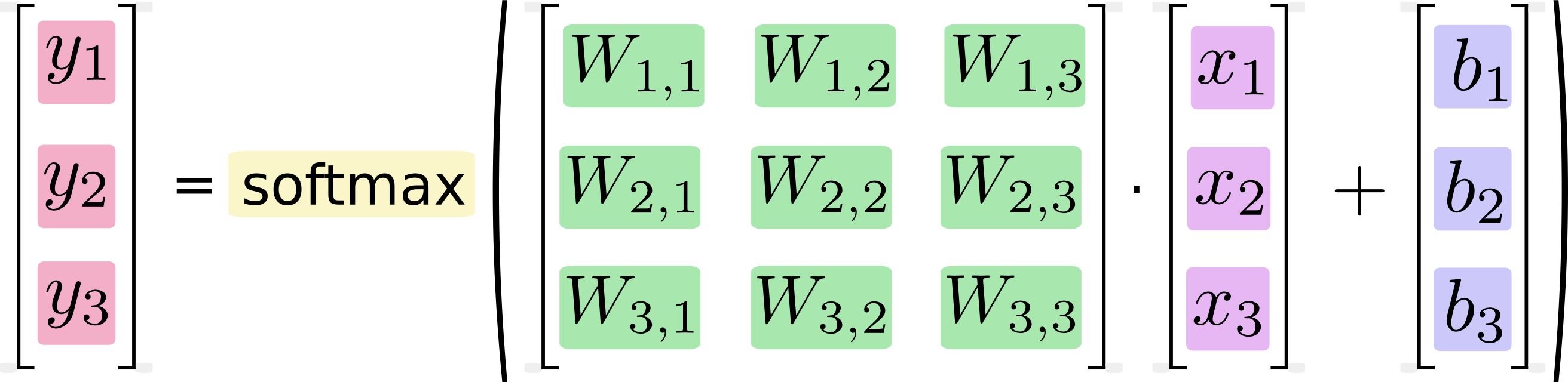## 2.4具体实现

``````import input_data #导入下载数据集的文件
import tensorflow as tf #导入TF

x = tf.placeholder("float", [None, 784])  #存储图片的可变长度变量
W = tf.Variable(tf.zeros([784,10])) #机器学习要完成的权重W变量而且初始化为0,因为这两个本来就是我们需要模型去学习训练出来的参数.
b = tf.Variable(tf.zeros()) #偏置量
y = tf.nn.softmax(tf.matmul(x,W) + b) #建立softmax模型,一句搞定,很简单
y_ = tf.placeholder("float", [None,10]) #真实标签数据
cross_entropy = -tf.reduce_sum(y_*tf.log(y))
init = tf.initialize_all_variables()
sess = tf.Session()
sess.run(init)
for i in range(1000):
batch_xs, batch_ys = mnist.train.next_batch(100)
sess.run(train_step, feed_dict={x: batch_xs, y_: batch_ys})
correct_prediction = tf.equal(tf.argmax(y,1), tf.argmax(y_,1))
accuracy = tf.reduce_mean(tf.cast(correct_prediction, "float"))
print sess.run(accuracy, feed_dict={x: mnist.test.images, y_: mnist.test.labels})
``````

### 2.4.1学习方法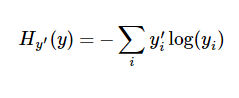### 2.4.2评估方法

correct_prediction = tf.equal(tf.argmax(y,1), tf.argmax(y_,1))

accuracy = tf.reduce_mean(tf.cast(correct_prediction, “float”))

### 2.4.3运行代码

``````python main.py
````````````python fully_connected_feed.py
``````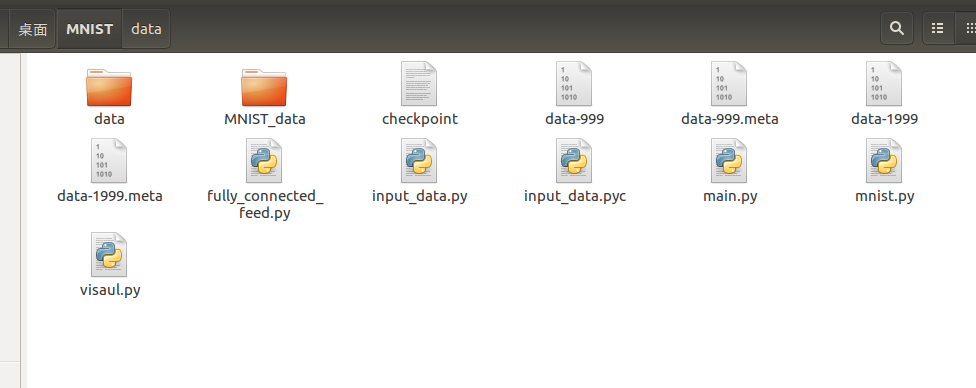``````sudo pip install --upgrade tensorflow
``````

``````tensorboard --logdir=/home/way/桌面/MNIST
``````